Python package for concise, transparent, and accurate predictive modeling. All sklearn-compatible and easy to use.imodels overview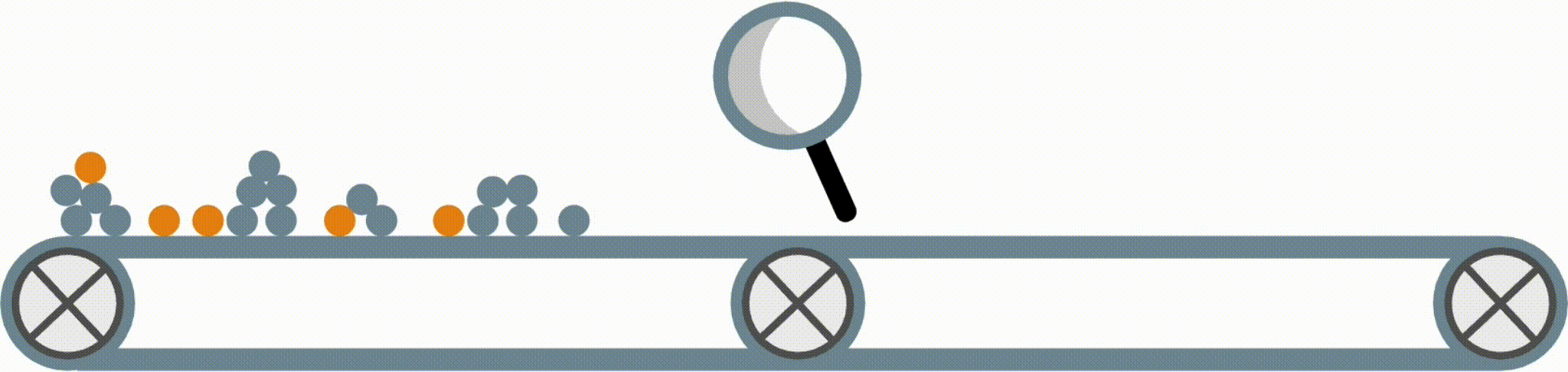Modern machine-learning models are increasingly complex, often making them difficult to interpret. This package provides a simple interface for fitting and using state-of-the-art interpretable models, all compatible with scikit-learn. These models can often replace black-box models (e.g. random forests) with simpler models (e.g. rule lists) while improving interpretability and computational efficiency, all without sacrificing predictive accuracy! Simply import a classifier or regressor and use the fit and predict methods, same as standard scikit-learn models.

from imodels import BoostedRulesClassifier, BayesianRuleListClassifier, GreedyRuleListClassifier, SkopeRulesClassifier # see more models below
from imodels import SLIMRegressor, RuleFitRegressor

model = BoostedRulesClassifier()  # initialize a model
model.fit(X_train, y_train)   # fit model
preds = model.predict(X_test) # discrete predictions: shape is (n_test, 1)
preds_proba = model.predict_proba(X_test) # predicted probabilities: shape is (n_test, n_classes)
print(model) # print the rule-based model

-----------------------------
# the model consists of the following 3 rules
# if X1 > 5: then 80.5% risk
# else if X2 > 5: then 40% risk
# else: 10% risk

Installation

Install with pip install imodels (see here for help).

Supported models

Model Reference Description
Rulefit rule set , , Fits a sparse linear model on rules extracted from decision trees
Skope rule set , Extracts rules from gradient-boosted trees, deduplicates them,
then linearly combines them based on their OOB precision
Boosted rule set , , Sequentially fits a set of rules with Adaboost
Slipper rule set , Sequentially learns a set of rules with SLIPPER
Bayesian rule set , , Finds concise rule set with Bayesian sampling (slow)
Optimal rule list , , Fits rule list using global optimization for sparsity (CORELS)
Bayesian rule list , , Fits compact rule list distribution with Bayesian sampling (slow)
Greedy rule list , Uses CART to fit a list (only a single path), rather than a tree
OneR rule list , Fits rule list restricted to only one feature
Optimal rule tree , , Fits succinct tree using global optimization for sparsity (GOSDT)
Greedy rule tree , , Greedily fits tree using CART
C4.5 rule tree , , Greedily fits tree using C4.5
Iterative random
forest
, , Repeatedly fit random forest, giving features with
high importance a higher chance of being selected
Sparse integer
linear model
, Sparse linear model with integer coefficients
Sapling Sums , Sum of small trees with very few total rules (SAPS)
Shrunk trees , Use regularization to improve trees
More models (Coming soon!) Lightweight Rule Induction, MLRules, ...

Docs , Reference code implementation , Research paper

Also see our util functions for downloading popular tabular datasets (e.g. compas). These functions, in conjunction with imodels-data and imodels-experiments, make it simple to download data and run experiments on new models.
Also see our simple function for explaining classification errors. Fit an interpretable model to explain a previous model's errors (ex. in this notebook).
Also see our fast and effective discretizers for data preprocessing.
Discretizer Reference Description
MDLP , , Discretize using entropy minimization heuristic
Simple , Simple KBins discretization
Random Forest Discretize into bins based on random forest split popularity

The final form of the above models takes one of the following forms, which aim to be simultaneously simple to understand and highly predictive:

Rule set Rule list Rule tree Algebraic models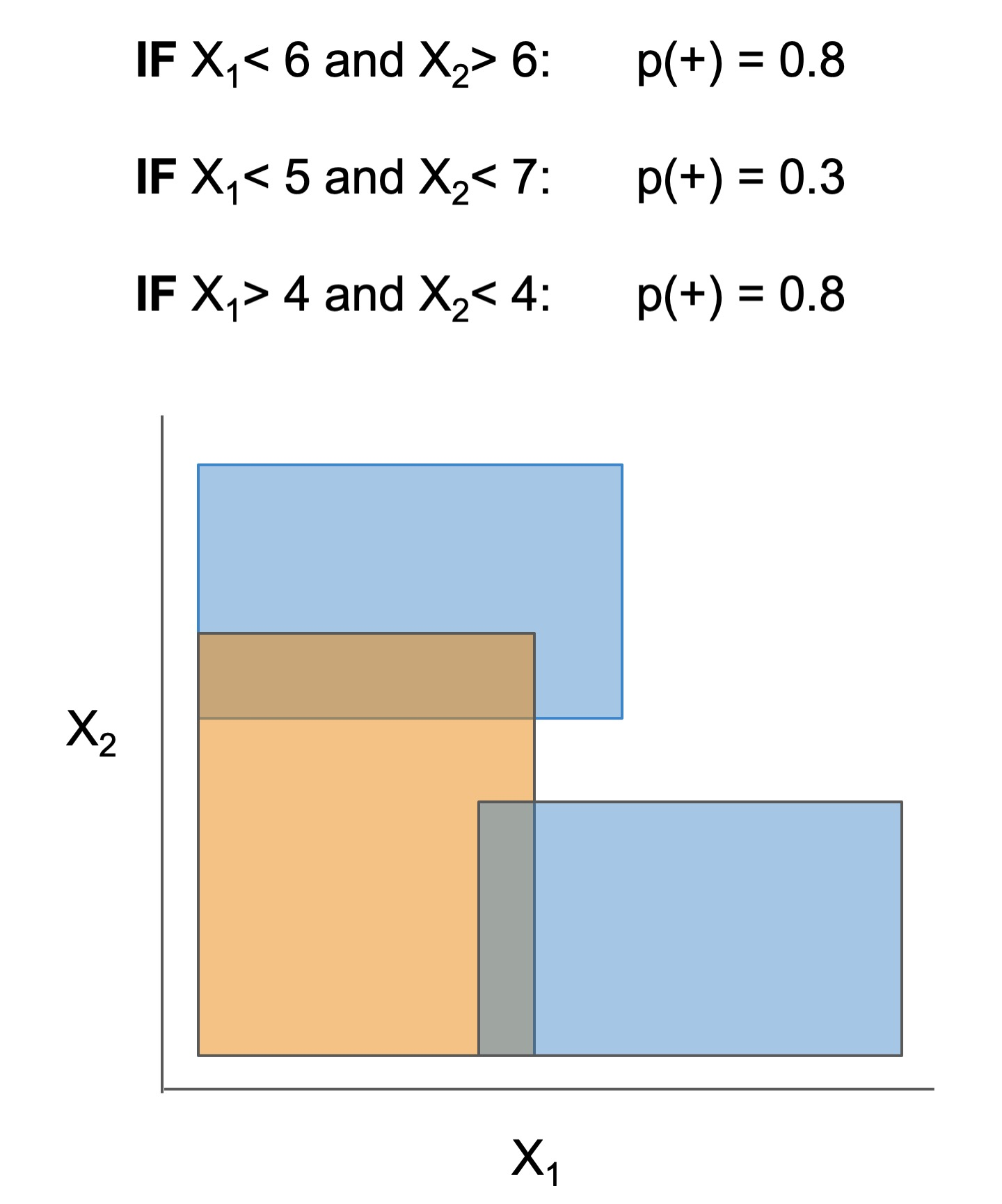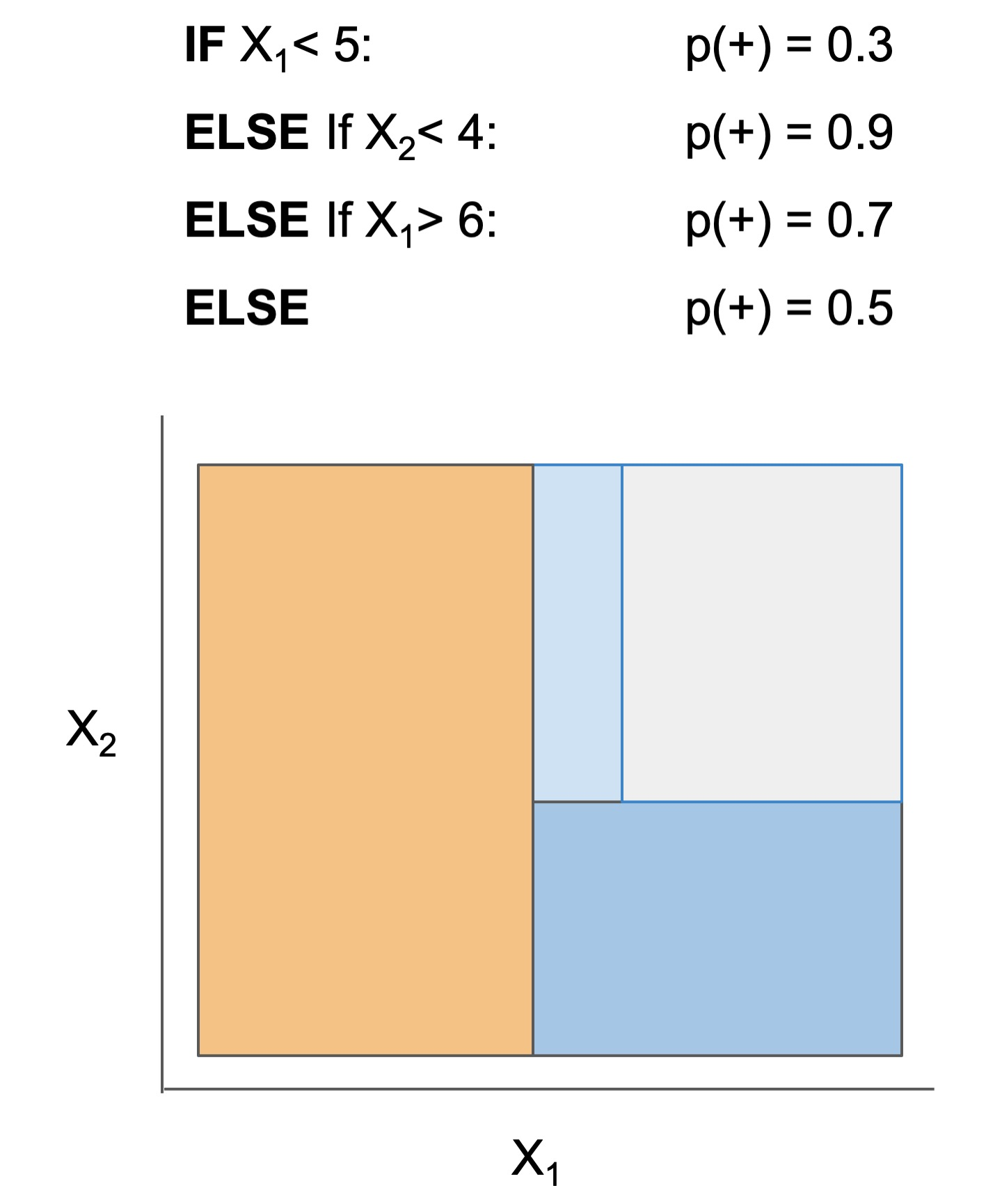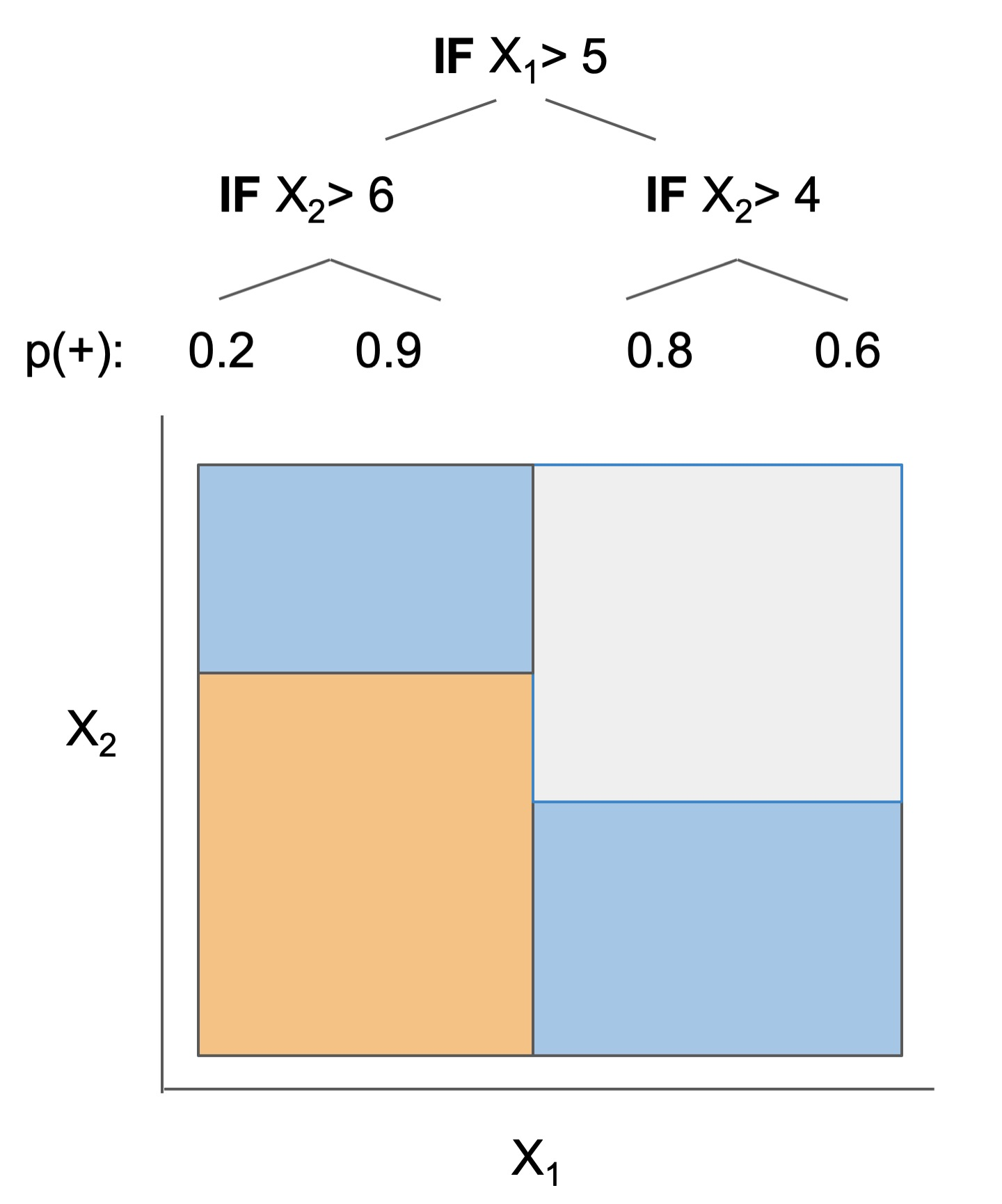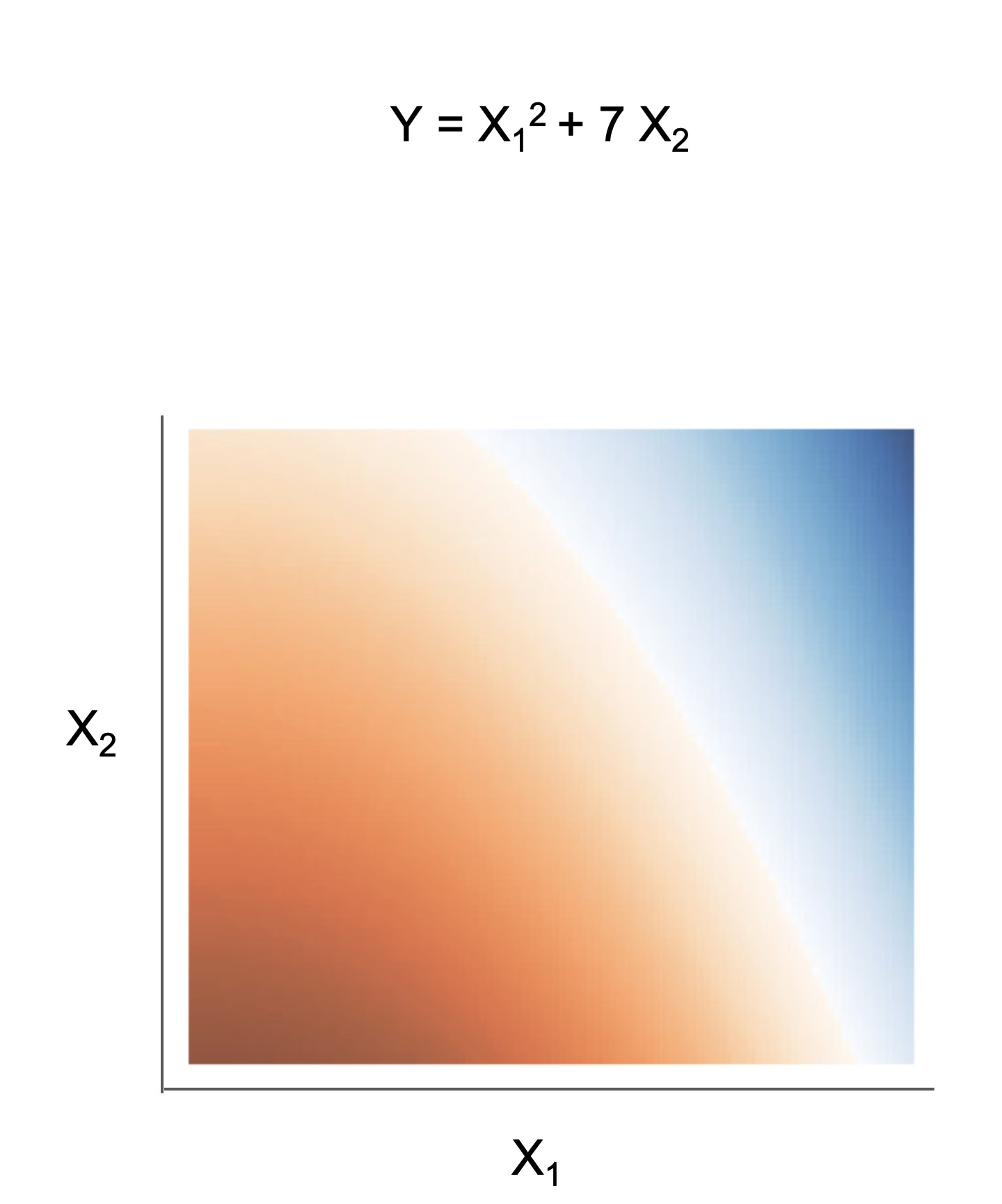Different models and algorithms vary not only in their final form but also in different choices made during modeling. In particular, many models differ in the 3 steps given by the table below.

ex. RuleFit and SkopeRules RuleFit and SkopeRules differ only in the way they prune rules: RuleFit uses a linear model whereas SkopeRules heuristically deduplicates rules sharing overlap.
ex. Bayesian rule lists and greedy rule lists Bayesian rule lists and greedy rule lists differ in how they select rules; bayesian rule lists perform a global optimization over possible rule lists while Greedy rule lists pick splits sequentially to maximize a given criterion.
ex. FPSkope and SkopeRules FPSkope and SkopeRules differ only in the way they generate candidate rules: FPSkope uses FPgrowth whereas SkopeRules extracts rules from decision trees.

See the docs for individual models for futher descriptions.

Rule candidate generation Rule selection Rule postprocessing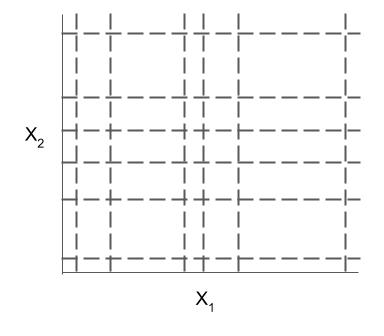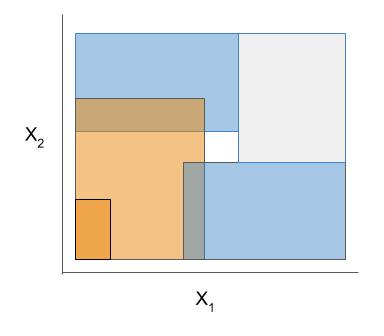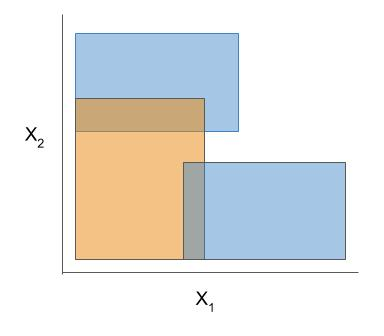The code here contains many useful and customizable functions for rule-based learning in the util folder. This includes functions / classes for rule deduplication, rule screening, and converting between trees, rulesets, and neural networks.

Demo notebooks

Demos are contained in the notebooks folder.

imodels demo Shows how to fit, predict, and visualize with different interpretable models
imodels colab demoShows how to fit, predict, and visualize with different interpretable models
clinical decision rule notebook Shows an example of using imodels for deriving a clinical decision rule
posthoc analysis We also include some demos of posthoc analysis, which occurs after fitting models: posthoc.ipynb shows different simple analyses to interpret a trained model and uncertainty.ipynb contains basic code to get uncertainty estimates for a model

Different models support different machine-learning tasks. Current support for different models is given below (each of these models can be imported directly from imodels (e.g. from imodels import RuleFitClassifier):

Model Binary classification Regression Notes
Rulefit rule set RuleFitClassifier RuleFitRegressor
Skope rule set SkopeRulesClassifier
Boosted rule set BoostedRulesClassifier
SLIPPER rule set SlipperClassifier
Bayesian rule set BayesianRuleSetClassifier Fails for large problems
Optimal rule list (CORELS) OptimalRuleListClassifier Requires corels, fails for large problems
Bayesian rule list BayesianRuleListClassifier
Greedy rule list GreedyRuleListClassifier
OneR rule list OneRClassifier
Optimal rule tree (GOSDT) OptimalTreeClassifier Requires gosdt, fails for large problems
Greedy rule tree (CART) GreedyTreeClassifier GreedyTreeRegressor
C4.5 rule tree C45TreeClassifier
Iterative random forest IRFClassifier Requires irf
Sparse integer linear model SLIMClassifier SLIMRegressor Requires extra dependencies for speed
Sapling Sums (SAPS) SaplingSumClassifier SaplingSumRegressor
Shrunk trees ShrunkTreeClassifierCV ShrunkTreeRegressorCV Wraps any sklearn tree-based model

References

• Interpretable ML good quick overview: murdoch et al. 2019, pdf
• Interpretable ML book: molnar 2019, pdf
• Case for interpretable models rather than post-hoc explanation: rudin 2019, pdf
• Review on evaluating interpretability: doshi-velez & kim 2017, pdf
Reference implementations (also linked above) The code here heavily derives from the wonderful work of previous projects. We seek to to extract out, unify, and maintain key parts of these projects.
Related packages
• gplearn: symbolic regression/classification
• pysr: fast symbolic regression
• interpretml: boosting-based gam
• h20 ai: gams + glms (and more)
• optbinning: data discretization / scoring models
• For updates, star the repo, see this related repo, or follow @csinva_
• Please make sure to give authors of original methods / base implementations appropriate credit!
• Contributing: pull requests very welcome!

If it's useful for you, please star/cite the package, and make sure to give authors of original methods / base implementations credit:

@software{
imodels2021,
title        = {{imodels: a python package for fitting interpretable models}},
journal      = {Journal of Open Source Software}
publisher    = {The Open Journal},
year         = {2021},
author       = {Singh, Chandan and Nasseri, Keyan and Tan, Yan Shuo and Tang, Tiffany and Yu, Bin},
volume       = {6},
number       = {61},
pages        = {3192},
doi          = {10.21105/joss.03192},
url          = {https://doi.org/10.21105/joss.03192},
}

Get A Weekly Email With Trending Projects For These Topics
No Spam. Unsubscribe easily at any time.
Python (1,140,932
Jupyter Notebook (241,107
Machine Learning (31,673
Data Science (9,156
Artificial Intelligence (5,554
Ai (4,798
Statistics (4,327
Scikit Learn (1,987
Ml (1,563
Supervised Learning (623
Rules (408
Explainable Ai (256
Interpretability (231
Explainable Ml (102
Imodel (14
Rule Learning (4
Optimal Classification Tree (2
Related Projects